• 对外差激光干涉仪中的非线性误差理论进行了综述，着重分析了一阶、二阶非线性误差的形成机理、非线性误差的大小及表现形式，并对非线性误差检测和补偿方法的研究进展进行了详细阐述和分析，指出了各类方法的应用特点...
• 为了补偿激光外差干涉纳米测量中的非线性误差,提出了一种减小非线性误差的一次谐波方法。基于全反射理论分析了镀膜实体角锥棱镜反射光偏振特性,并由此推导出角锥棱镜反射光偏振特性及测量角锥棱镜以其运动方向为轴线...
• 仪器误差可以分为原理误差、制造误差和运行误差三大类。 　（1）原理误差 　原理误差由于理论不完善或采取近似理论而产生。它与制造精度无关，而是由设计决定。仪器采取何种方案，进行何种近似处理，便会产生何...
• 提出了压电陶瓷驱动器的移相误差和光电探测器的非线性误差相互抵消的误差补偿技术,并给出了判别误差匹配有效性的实用判据。仿真表明,这种误差补偿技术可以使测量误差减小约一个量级。证明了在不考虑波前幅度信息时,...
• 仪器误差来源可以分为原理误差、制造误差和运行误差三大类。 　（1）原理误差 　原理误差由于理论不完善或采取近似理论而产生。它与制造精度无关，而是由设计决定。仪器采取何种方案，进行何种近似处理，便会...
• 智能仪器-第四章-基础数据处理算法与软测量技术 系统非线性校正 智能仪器 第3版 吉林大学 程德福、林君 机械工业出版社 第124页 表4-1 对表格中数据用曲线拟合法，连续函数直线拟合，进行非线性校正。并指出最大误...
智能仪器-第四章-基础数据处理算法与软测量技术
系统非线性校正

智能仪器 第3版 吉林大学 程德福、林君 机械工业出版社 第124页 表4-1

对表格中数据用曲线拟合法，连续函数直线拟合，进行非线性校正。并指出最大误差点。
from functools import reduce
import numpy
import matplotlib.pyplot as plt
x = [0, 0.4, 0.8, 1.2, 1.61, 2.02, 2.44, 2.85, 3.27, 3.68, 4.1, 4.51, 4.92, 5.33, 5.73, 6.14, 6.54,
6.94, 7.34, 7.74, 8.14, 8.54, 8.94, 9.34, 9.75, 10.15, 10.56, 10.97, 11.38, 11.8, 12.21, 12.62,
13.04, 13.46, 13.87, 14.29, 14.71, 15.13, 15.55, 15.97, 16.4, 16.82, 17.24, 17.67, 18.09,
18.51, 18.94, 19.36, 19.79, 20.21]
y = range(0,500,10)
A = sum(x)     #x的和
B = reduce(lambda x, y: x + y, (map(lambda x: x ** 2, x)))     #x的平方和
C = sum(y)     #y的和
D = sum([a*b for a,b in zip(x,y)])      #x*y的和
E = numpy.array([[50,A],[A,B]])
F = numpy.array([[C],[D]])
[a0,a1] = numpy.linalg.solve(E,F)       #linalg线性代数包，solve求矩阵函数E*[a0,a1]=Y
Y = a0+a1*x
error = Y-y
v=max(error)            #最大误差
print(a0,a1)
print(v)

#作图
plt.title('0-490°C的镍铬-镍铬热电偶分度图')  # 折线图标题
plt.rcParams['font.sans-serif'] = ['SimHei']  # 显示汉字
plt.xlabel('温度/°C')  # x轴标题
plt.ylabel('电动势/mV')  # y轴标题
plt.plot(x, y)  # 绘制折线图
plt.plot(x, Y)
plt.legend(['真实值', '预测值'])  # 设置折线名称
plt.show()  # 显示折线图

分度图如下：展开全文python numpy 矩阵 plt
• 共光路外差干涉仪具有很高的分辨率,但因为安装、调试误差会产生非线性误差,影响系统的测量精度。着重分析了在共光路外差干涉仪中由激光光源的椭圆偏振化和沃拉斯顿棱镜的安装方位角误差同时存在的情况下,引起的频率...
• 介绍了虚拟仪器系统信号传递过程中引进的各类误差，...还研究了虚拟仪器系统误差补偿和修正方法，即利用软件来修正和补偿系统误差，特别是非线性误差，并应用于虚拟式噪声分析仪的误差修正，获得了高精度的测量结果。
• 针对虚拟仪器系统存在的非线性动态测量误差,提出了一种新的补偿方法。该方法依据虚拟仪器系统的静态和动态标定数据,采用最小二乘支持向量机...实验结果表明该方法用于虚拟仪器系统动态非线性误差补偿的有效性及优越性。
• 测试系统中任何额外产生的电流都会加到被测电流中去而引起误差。这种电流可以在内部产生，如仪器的输入偏置电流；也可以从外部而来，如来自绝缘子和电缆。以下将讨论各种电流产生原因。 　1. 偏置电流 　偏置电流...
• 静态特性是表示传感器在被测输入量的各个值处于稳定状态时的输入—输出关系，更能突出反映传感器的各项指标，传感器静态特性主要指线性度、迟后性、重复性和静态误差。以往对传感器静态特性的测试、分析需要多种仪器...
        在测量仪器上经常会用到线性拟合，通过测量的标准仪器标定出几个点，写进mcu的flash里面，比如说采到adc的值500对应标准盐溶液浓度的10%，1000对应盐溶液浓度20%，如果测量得知中间的值都是线性的，那么adc采到的值750，就对应浓度15%。
线性拟合分为两种情况，一种是整个量程线性度都非常的好，那么只需计算出一个k值就好，然后其他的点根据公式就可以计算出来，二是整个量程线性度不好，需要分段计算k值，那么这就需要多段线性拟合。线性相关的程度可以通过Matalab的curvefiting工具来帮你自动计算出k值，然后看rms的大小表示出的相关度的大小，也就是线性拟合出来的误差。这个curvefiting的使用后面我电脑装上Matalab再在这里补充上，这个工具确实很重要的。
1、整个量程线性度都很好的情况。
以我之前做过的一个激光测距仪的温度补偿为例，首先以室温25℃测出的距离为标准，温度升高距离增加，温度降低距离减小，比如测得数据如下：
10℃     900mm
15℃     902mm
20℃     904mm
25℃     906mm
30℃     908mm
35℃     910mm
40℃     912mm
从数据可以看出温度变化5℃时，距离也会增加或者减小2mm，也就是k=2/5=0.4，那么首先机器在室温标准距离时就需要记录标定时的温度t，然后补偿的时候，根据当时的温度与标定时的温度做差，例如当前温度是32℃，标准时的温度是25℃，那么相减就是7摄氏度，然后再乘以0.4，就是变化2.8mm，所以最终的距离就是906mm+2.8mm=908.8mm。实际中的k值计算可以直接通过Matalab的curvefitting工具自动计算出来的。
2、多段线性拟合
原理和多段拟合差不多，原因是从曲线看，不同量程段的线性度有差异的，下面我贴一下我的多选线性拟合的代码：
u16 Fitting_Curve(u32 Num)//多段线性拟合
{
static u8 number;
double Kr;
double Br;
u16 Result = 0;

static u16 Result_Current = 0;
u16 number_2[Numr] = {0,     100,    300,   500,   700,   900,  1200,  1400,  1500};

for( number=1; number<Numr; number++)
{
if(Num<Data_buff[number])
{
break;
}
}

if(number >= Numr)
number = Numr-1;

Kr = (number_2[number] - number_2[number-1]);
Kr = Kr/(Data_buff[number] - Data_buff[number-1]);
Br = (number_2[number-1] - Kr * Data_buff[number-1]);
_main_pro.salinity_double = Kr * Num + Br;

Result = (u16)_main_pro.salinity_double;

/*	if((Result%10)<5)
Result = Result/10;
else
Result = Result/10 + 1;

if((Result_Current - Result) * (Result_Current - Result) >= 4)
{
Result_Current = Result;
}
else
{
Result = Result_Current;
}

*/
return Result;
}

展开全文• 线性回归 假设Linear Regression is the bicycle of regression models. It’s simple yet incredibly useful. It can be used in a variety of domains. It has a nice closed formed solution, which makes model ...

线性回归 假设

Linear Regression is the bicycle of regression models. It’s simple yet incredibly useful. It can be used in a variety of domains. It has a nice closed formed solution, which makes model training a super-fast non-iterative process.
线性回归是回归模型的基础。 这很简单，但却非常有用。 它可以用于多种领域。 它具有良好的封闭式解决方案，这使得模型训练成为超快速的非迭代过程。
A Linear Regression model’s performance characteristics are well understood and backed by decades of rigorous research. The model’s predictions are easy to understand, easy to explain and easy to defend.
线性回归模型的性能特征已得到数十年的严格研究的很好理解和支持。 该模型的预测易于理解，易于解释和易于捍卫。

If there only one regression model that you have time to learn inside-out, it should be the Linear Regression model.
如果只有一个回归模型可供您内外学习，则应该使用线性回归模型。

If your data satisfies the assumptions that the Linear Regression model, specifically the Ordinary Least Squares Regression (OLSR) model makes, in most cases you need look no further.
如果您的数据满足线性回归模型(特别是普通最小二乘回归(OLSR)模型)所做的假设，则在大多数情况下，您无需再进行任何研究。
Which brings us to the following four assumptions that the OLSR model makes:
这使我们得出OLSR模型做出的以下四个假设：
Linear functional form: The response variable y should be a linearly related to the explanatory variables X. 线性函数形式：响应变量y应该与解释变量X线性相关。 Residual errors should be i.i.d.: After fitting the model on the training data set, the residual errors of the model should be independent and identically distributed random variables. 残留误差应该被消除：将模型拟合到训练数据集之后，模型的残留误差应该是独立的并且分布均匀的随机变量。 Residual errors should be normally distributed: The residual errors should be normally distributed. 残留误差应呈正态分布：残留误差应呈正态分布。 Residual errors should be homoscedastic: The residual errors should have constant variance. 残留误差应为等方差：残留误差应具有恒定的方差。
Let’s look at the four assumptions in detail and how to test them.
让我们详细看一下这四个假设以及如何测试它们。
假设1：线性函数形式 (Assumption 1: Linear functional form)
Linearity requires little explanation. After all, if you have chosen to do Linear Regression, you are assuming that the underlying data exhibits linear relationships, specifically the following linear relationship:
线性几乎不需要解释。 毕竟，如果您选择进行线性回归，则假定基础数据具有线性关系，特别是以下线性关系：
y = β*X + ϵ
y = β * X + ϵ
Where y is the dependent variable vector, X is the matrix of explanatory variables which includes the intercept, β is the vector of regression coefficients and ϵ is the vector of error terms i.e. the portion of y that X is unable to explain.
其中y是因变量矢量， X是解释变量的矩阵，其中包括截距， β是回归系数的向量， ϵ是误差项的向量，即y中X不能解释的部分。
How to test the linearity assumption using Python
如何使用Python测试线性假设
This can be done in two ways:
这可以通过两种方式完成：
An easy way is to plot y against each explanatory variable x_j and visually inspect the scatter plot for signs of non-linearity. 一种简单的方法是针对每个解释变量x_j绘制y并目视检查散点图是否存在非线性迹象。 One could also use the DataFrame.corr() method in Pandas to get the Pearson’s correlation coefficient ‘r’ between the response variable y and each explanatory variable x_j to get a quantitative feel for the degree of linear correlation. 还可以在Pandas中使用DataFrame.corr()方法来获得响应变量y与每个解释变量x_j之间的皮尔逊相关系数'r' ，从而获得线性相关程度的定量感觉。

Note that Pearson’s ‘r’ should be used only when the the relation between y and X is known to be linear.
请注意，仅当已知y和X之间的关系为线性时，才应使用Pearson的“ r”。

Let’s test the linearity assumption on the following data set of 9568 observations of 4 operating parameters of a combined cycle power plant taken over 6 years:
让我们根据以下6组观察结果得出的线性假设，这些数据是对联合循环发电厂在6年内进行的4个运行参数的9568个观测值的：
The explanatory variables x_j are as the following 4 power plant parameters:
说明变量x_j如下四个电厂参数：
Ambient_Temp in CelsiusExhaust_Volume in column height of Mercury in centimetersAmbient_Pressure in millibars of MercuryRelative_Humidity expressed as a percentage
Ambient_Temp摄氏Exhaust_Volume在厘米水银柱高度Ambient_Pressure水星Relative_Humidity的毫巴，以百分比表示
The response variable y is Power_Output of the power plant in MW.
响应变量y是发电厂的Power_Output ，单位为MW。
Let’s load the data set into a Pandas DataFrame.
让我们将数据集加载到Pandas DataFrame中。
import pandas as pdfrom patsy import dmatricesfrom matplotlib import pyplot as pltimport numpy as npdf = pd.read_csv('power_plant_output.csv', header=0)
Plot the scatter plots of each explanatory variable against the response variable Power_Output.
绘制每个解释变量相对于响应变量Power_Output的散点图。
df.plot.scatter(x='Ambient_Temp', y='Power_Output')plt.xlabel('Ambient_Temp', fontsize=18)plt.ylabel('Power_Output', fontsize=18)plt.show()df.plot.scatter(x='Exhaust_Volume', y='Power_Output')plt.xlabel('Exhaust_Volume', fontsize=18)plt.ylabel('Power_Output', fontsize=18)plt.show()df.plot.scatter(x='Ambient_Pressure', y='Power_Output')plt.xlabel('Ambient_Pressure', fontsize=18)plt.ylabel('Power_Output', fontsize=18)plt.show()df.plot.scatter(x='Relative_Humidity', y='Power_Output')plt.xlabel('Relative_Humidity', fontsize=18)plt.ylabel('Power_Output', fontsize=18)plt.show()
Here is a collage of the four plots:
这是四个情节的拼贴画：
Scatter plots of Power_Output against each explanatory variable

You can see that Ambient_Temp and Exhaust_Volume seem to be most linearly related to the power plant’s Power_Output, followed by Ambient_Pressure and Relative_Humidity in that order.
您可以看到，Ambient_Temp和Exhaust_Volume似乎与发电厂的Power_Output线性关系最大，其次是Ambient_Pressure和Relative_Humidity。
Let’s also print out the Pearson’s ‘r’:
让我们也打印出皮尔逊的“ r”：
df.corr()['Power_Output']
We get the following output, which backs up our visual intuition:
我们得到以下输出，它支持我们的视觉直觉：
Ambient_Temp        -0.948128Exhaust_Volume      -0.869780Ambient_Pressure     0.518429Relative_Humidity    0.389794Power_Output         1.000000Name: Power_Output, dtype: float64

Related read: The Intuition Behind Correlation, for an in-depth explanation of the Pearson’s correlation coefficient.
相关阅读： 相关性背后的直觉 ，深入了解皮尔逊相关系数。

假设2：iid残差 (Assumption 2: i.i.d. residual errors)
The second assumption that one makes while fitting OLSR models is that the residual errors left over from fitting the model to the data are independent, identically distributed random variables.
在拟合OLSR模型时做出的第二个假设是，将模型拟合到数据后剩下的残留误差是独立的 ， 均匀分布的 随机变量 。
We break this assumption into three parts:
我们将此假设分为三个部分：
The residual errors are random variables, 残留误差是随机变量， They are independent random variables, and 它们是独立的随机变量，并且 Their probability distributions are identical. 它们的概率分布是相同的 。
为什么残留误差是随机变量？ (Why are residual errors random variables?)
After we train a Linear Regression model on a data set, if we run the training data through the same model, the model will generate predictions. Let’s call them y_pred. For each predicted value y_pred in the vector y_pred, there is a corresponding actual value y from the response variable vector y. The difference (y — y_pred) is the residual error ‘ε’. There are as many of these ε as the number of rows in the training set and together they form the residual errors vector ε.
在数据集上训练线性回归模型后，如果通过同一模型运行训练数据，则该模型将生成预测。 我们称它们为y_pred。 在y_pred矢量y_pred各预测值，存在来自响应变量矢量y相应的实际值y。 差(y_y_pred)是残余误差“ ε” 。 这些ε与训练集中的行数一样多，它们一起形成了残留误差向量ε 。
Each residual error ε is a random variable. To understand why, recollect that our training set (y_train, X_train) is just a sample of n values drawn from some very large population of values.
每个残余误差ε是一个随机变量 。 要了解原因，请回想一下我们的训练集(y_train，X_train)只是从一些非常大的值总体中得出的n个值的样本 。
If we had drawn a different sample (y_train’, X_train’) from the same population, the model would have fitted somewhat differently on this second sample, thereby producing a different set of predictions y_pred’, and therefore a different set of residual errors ε = (y’ — y_pred’).
如果我们从同一总体中抽取了不同的样本(y_train'，X_train') ，则该模型在第二个样本上的拟合将有所不同，从而产生一组不同的预测y_pred' ，从而产生一组不同的残差ε = (y'— y_pred') 。
A third training sample drawn from the population would have, after training the model on it, generated a third set of residual errors ε = (y’’ — y_pred’’), and so on.
在对模型进行训练之后，从总体中提取的第三次训练样本将产生第三组残差误差ε=(y''-y_pred'')，依此类推。
One can now see how each residual error in the vector ε can take a random value from as many set of values as the number of sample training data sets one is willing to train the model on, thereby making each residual error ε a random variable.
现在可以看到向量ε中的每个残留误差   可以从一个愿意训练模型的样本训练数据集的数量中选取一个任意值作为随机值，从而使每个残差ε   一个随机变量。
Why do residual errors need to be independent?
为什么残留误差需要独立？
Two random variables are independent if the probability of one of them taking up some value doesn’t depend on what value the other variable has taken. When you roll a die twice, the probability of its coming up as one, two,…,six in the second throw does not depend on the value it came up on the first throw. So the two throws are independent random variables that can each take a value of 1 thru 6 independent of the other throw.
如果两个随机变量占据一个值的概率不取决于另一个变量取什么值，则两个变量是独立的。 当您掷骰子两次时，其在第二次掷骰中出现的概率为一，二，...，六，这并不取决于它在第一掷掷骰中获得的值。 因此，两次抛出是独立的随机变量，可以独立于另一次抛出而分别取值1到6。
In the context of regression, we have seen why the residual errors of the regression model are random variables. If the residual errors are not independent, they will likely demonstrate some sort of a pattern (which is not always obvious to the naked eye). There is information in this pattern that the regression model wasn’t able to capture during its training on the training set, thereby making the model sub-optimal.
在回归的背景下，我们已经看到了为什么回归模型的残差是随机变量。 如果残留误差不是独立的，则它们可能会显示出某种模式(肉眼并不总是很明显)。 在这种模式下，有信息表明，回归模型在训练集上的训练过程中无法捕获，因此使模型次优。
If the residual errors aren’t independent, it may mean a number of things:
如果残留错误不是独立的，则可能意味着很多事情：
One or more important explanatory variables are missing from your model. The effect of the missing variables is showing through as a pattern in the residual errors. 您的模型中缺少一个或多个重要的解释变量。 遗漏变量的影响通过模式显示在残余误差中。 The linear model you have built is just the wrong kind of model for the data set. For e.g. if the data set shows obvious non-linearity and you try to fit a linear regression model on such a data set, the nonlinear relationships between y and X will show through in the residual errors of regression in the form of a distinct pattern. 您建立的线性模型只是错误的数据集模型。 例如，如果数据集显示出明显的非线性，并且您尝试在此类数据集上拟合线性回归模型，则y和X之间的非线性关系将以不同模式的形式显示在回归的残留误差中。 A third interesting cause of non-independence of residual errors is what’s known as multicolinearity which means that the explanatory variables are themselves linearly related to each other. Multicolinearity causes the model’s coefficients to become unstable, i.e. they will swing wildly from one training run to next when trained on different training sets. This can make the model’s overall goodness-of-fit statistics questionable. Another serious effect of multicoliearity, especially extreme multicolinearity, is that tha model’s least squares solver may throw up infinities during the model fitting process thereby making it impossible to fit the model on the training data. 残余误差不独立的第三个有趣原因是所谓的多重共线性 ，这意味着解释变量本身彼此之间呈线性关系。 多重共线性导致模型的系数变得不稳定，即，当在不同的训练集上训练时，它们将从一次训练奔跑到下一次训练。 这可能会使模型的整体拟合优度统计数据令人怀疑。 多共线性(尤其是极端多线性)的另一个严重影响是，模型的最小二乘法求解器可能会在模型拟合过程中抛出无穷大，从而无法将模型拟合到训练数据上。
How to test for independence of residual errors?
如何测试残差的独立性？
It’s not easy to verify independence. But sometimes one can detect patterns in the plot of residual errors versus the predicted values or the plot of residual errors versus actual values.
验证独立性并不容易。 但是有时人们可以在残留误差与预测值的关系图或残留误差与实际值的 关系图中检测模式。
Another common technique is to use the Dubin-Watson test which measures the degree of correlation of each residual error with the ‘previous’ residual error. This is known as lag-1 auto-correlation and it is a useful technique to find out if residual errors of a time series regression model are independent.
另一种常见的技术是使用Dubin-Watson检验 ，该检验测量每个残差与“先前”残差的相关程度。 这被称为lag-1自相关 ，它是一种有用的技术，可用来确定时间序列回归模型的残差是否独立。
Let’s fit a linear regression model to the Power Plant data and inspect the residual errors of regression.
让我们将线性回归模型拟合到电厂数据并检查回归的残留误差。
We’ll start by creating the model expression using the Patsy library as follows:
我们将从使用Patsy库创建模型表达式开始，如下所示：
model_expr = 'Power_Output ~ Ambient_Temp + Exhaust_Volume + Ambient_Pressure + Relative_Humidity'
In the above model expression, we are telling Patsy that Power_Output is the response variable while Ambient_Temp, Exhaust_Volume, Ambient_Pressure and Relative_Humidity are the explanatory variables. Patsy will add the regression intercept by default.
在上面的模型表达式中，我们告诉Patsy，Power_Output是响应变量，而Ambient_Temp，Exhaust_Volume，Ambient_Pressure和Relative_Humidity是解释变量。 Patsy将默认添加回归截距。
We’ll use patsy to carve out the y and X matrices as follows:
我们将使用patsy来划分y和X矩阵，如下所示：
y, X = dmatrices(model_expr, df, return_type='dataframe')
Let’s also carve out the train and test data sets. The training data set will be 80% of the size of the overall (y, X) and the rest will be the testing data set:
让我们也分析一下训练和测试数据集。 训练数据集将是整体( y，X )大小的80％，其余的将是测试数据集：
mask = np.random.rand(len(X)) < 0.8X_train = X[mask]y_train = y[mask]X_test = X[~mask]y_test = y[~mask]
Finally, build and train an Ordinary Least Squares Regression Model on the training data and print the model summary:
最后，在训练数据上构建和训练普通最小二乘回归模型并打印模型摘要：
olsr_results = linear_model.OLS(y_train, X_train).fit()print('Training completed')print(olsr_results.summary())
We get the following output:
我们得到以下输出：
OLS Regression output printed out by statsmodels

Next, let’s get the predictions of the model on test data set and get its predictions:
接下来，让我们在测试数据集上获得模型的预测并获得其预测：
olsr_predictions = olsr_results.get_prediction(X_test)
olsr_predictions is of type statsmodels.regression._prediction.PredictionResult and the predictions can obtained from the PredictionResult.summary_frame() method:
olsr_predictions的类型为statsmodels.regression._prediction.PredictionResult，并且可以从PredictionResult.summary_frame()方法获得预测 ：
prediction_summary_frame = olsr_predictions.summary_frame()print(prediction_summary_frame)
The model’s predictions and the 95% lower and upper confidence levels

Let’s calculate the residual errors of regression ε = (y_test — y_pred):
让我们计算回归残差ε = (y_test — y_pred)：
resid = y_test['Power_Output'] - prediction_summary_frame['mean']
Finally, let’s plot resid against the predicted value y_pred=prediction_summary_frame[‘mean’]:
最后，让我们绘制resid   针对预测值y_pred=prediction_summary_frame['mean'] ：
plt.xlabel('Predicted Power Output', fontsize=18)plt.ylabel('Residual Error of Regression', fontsize=18)plt.scatter(y_test['Power_Output'], resid)plt.show()
We get the following plot:
我们得到以下图：
Residual errors plotted against the model’s predictions

One can see that the residuals are more or less pattern-less for smaller values of Power Output, but they seem to be showing a linear pattern at the higher end of the Power Output scale. It indicates that the model’s predictions at the higher end of the power output scale are less reliable than at the lower end of the scale.
可以看到，对于较小的Power Output值，残差或多或少地没有模式，但它们似乎在Power Output标度的高端显示线性模式。 它表明模型在功率输出比例尺高端的预测不如在尺度下端的可靠。
为什么残留错误应该具有相同的分布？ (Why should residual errors have Identical distributions?)
What identically distributed means is that residual error ε_i corresponding to the prediction for each data row, has the same probability distribution. If the distribution of errors is not identical, one cannot reliably use tests of significance such as the F-test for regression analysis or perform confidence interval testing on the predictions. Many of these tests depend on the residual errors being identically, and normally distributed. This brings us to the next assumption.
均匀分布的意思是与每个数据行的预测相对应的残留误差ε_i具有相同的概率分布。 如果误差的分布不相同，则无法可靠地使用重要性检验(例如F检验)进行回归分析或对预测进行置信区间检验。 这些测试中的许多测试都取决于残留误差是否相同且呈正态分布 。 这将我们带入下一个假设。
假设3：残留误差应正态分布 (Assumption 3: Residual errors should be normally distributed)
In the previous section, we saw how and why the residual errors of the regression are assumed to be independent, identically distributed (i.i.d.) random variables. Assumption 3 imposes an additional constraint. The errors should all have a normal distribution with a mean of zero. In statistical language:
在上一节中，我们了解了如何以及为什么将回归的残差误差假定为独立的，均匀分布的(iid)随机变量。 假设3施加了附加约束。 误差均应具有均值为零的正态分布。 用统计语言：

∀ i ∈ n, ε_i ~ N(0, σ²)
∀ 我 ∈N，ε_i〜N(0，σ²)

This notation is read as follows:
该符号的含义如下：
For all i in the data set of length n rows, the ith residual error of regression is a random variable that is normally distributed (that’s why the N() notation). This distribution has a mean of zero and a variance of σ². Furthermore, all ε_i have the same variance σ², i.e. they are identically distributed.
对于长度为n行的数据集中的所有i ，回归的第i个残差是一个正态分布的随机变量(这就是N ()表示法的原因)。 该分布的平均值为零，方差为σ²。 此外，所有ε_i具有相同的方差σ² ，即它们具有相同的分布。
It is a common misconception that linear regression models require the explanatory variables and the response variable to be normally distributed.
一个普遍的误解是，线性回归模型要求解释变量和响应变量呈正态分布。
More often than not, x_j and y will not even be identically distributed, leave alone normally distributed.
通常， x_j和y甚至不会均匀分布，更不用说正态分布了。

In Linear Regression, Normality is required only from the residual errors of the regression.
在线性回归中，仅从回归的残留误差中需要正态性。

In fact, normality of residual errors is not even strictly required. Nothing will go horribly wrong with your regression model if the residual errors ate not normally distributed. Normality is only a desirable property.
实际上，甚至没有严格要求残差的正态性。 如果残留误差的吃法不是正态分布的，那么您的回归模型将不会出现任何可怕的错误。 常态只是一个理想的属性。
What’s normally is telling you is that most of the prediction errors from your model are zero or close to zero and large errors are much less frequent than the small errors.
通常告诉您的是，模型中的大多数预测误差为零或接近零，大误差的发生频率远小于小误差。
如果残留误差未分配N(0，σ²)，会发生什么？ (What happens if the residual errors are not N(0, σ²) distributed?)
If the residual errors of regression are not N(0, σ²), then statistical tests of significance that depend on the errors having an N(0, σ²) distribution, simply stop working.
如果回归的残留误差不是N(0，σ²) ，则根据具有N(0，σ²)分布的误差的显着性统计检验，只需停止工作即可。
For example,
例如，
The F-statistic used by the F-test for regression analysis has the required Chi-squared distribution only if the regression errors are N(0, σ²) distributed. If regression errors are not normally distributed, the F-test cannot be used to determine if the model’s regression coefficients are jointly significant. You will then have to use some other test to figure out if your regression model did a better job than a straight line through the data set mean. F 检验用于回归分析的F统计量   仅当回归误差为N(0，σ²)分布时，才具有所需的卡方分布。 如果回归误差不是正态分布的，则F检验不能用于确定模型的回归系数是否共同显着。 然后，您将不得不使用其他测试来确定您的回归模型是否比通过数据集平均值的直线做得更好。 Similarly, the computation of t-values and confidence intervals assumes that regression errors are N(0, σ²) distributed. If the regression errors are not normally distributed, t-values for the model’s coefficients and the model’s predictions become inaccurate and you should not put too much faith into the confidence intervals for the coefficients or the predictions. 类似地， t值和置信区间的计算假定回归误差为N(0，σ²)分布。 如果回归误差不是正态分布的，则模型系数的t值和模型的预测将变得不准确，并且您不应在系数或预测的置信区间中过分置信。
A special case of non-normality: bimodally distributed residual errors
非正态的特殊情况：双峰分布残差
Sometimes, one finds that the model’s residual errors have a bimodal distribution i.e. they have two peaks. This may point to a badly specified model or a crucial explanatory variable that is missing from the model.
有时，人们发现模型的残留误差具有双峰分布，即它们具有两个峰值。 这可能表明模型指定不正确或模型中缺少关键的解释变量。
For example, consider the following situation:
例如，请考虑以下情况：
Your dependent variable is a binary variable such as Won (encoded as 1.0) or Lost (encoded as 0.0). But your linear regression model is giong to generate predictions on the continuous real number scale. If the model generates most of its predictions along a narrow range of this scale around 0.5, for e.g. 0.55, 0.58, 0.6, 0.61, etc. the regression errors will peak either on one side of zero (when the true value is 0), or on the other side of zero (when the true value is 1). This is a sign that your model is not able to decide whether the output should be 1 or 0, so it’s predicting a value that is around the average of 1 and 0.
您的因变量是一个二进制变量，例如Won(编码为1.0)或Lost(编码为0.0)。 但是您的线性回归模型可以在连续实数范围内生成预测。 如果模型在0.5左右的狭窄范围(例如0.55、0.58、0.6、0.61等)上生成大部分预测，则回归误差将在零的一侧达到峰值(当真实值为0时)，或在零的另一侧(当真实值为1时)。 这表明您的模型无法决定输出应为1还是0，因此它预测的值约为1和0的平均值。
This can happen if you are missing a key binary variable, known as an indicator variable, which influences the output value in the following way:
如果缺少关键的二进制变量(称为指示符变量)，则会以以下方式影响输出值：
When the variable’s value is 0, the output ranges within a certain range, say close to 0.
当变量的值为0时，输出范围在一定范围内，例如接近0。
When the variable’s value is 1, the output takes on a whole new range of values that are not there in the earlier range, say around 1.0.
当变量的值为1时，输出将采用一个较新的值范围，该范围不在较早的范围内，例如1.0。
If this variable is missing in your model, the predicted value will average out between the two ranges, leading to two peaks in the regression errors. Once this variable is added, the model is well specified, and it will correctly differentiate between the two possible ranges of the explanatory variable.
如果模型中缺少此变量，则预测值将在两个范围之间求平均值，从而导致回归误差出现两个峰值。 一旦添加了此变量，就可以很好地指定模型，并且可以正确地区分解释变量的两个可能范围。
Related read: When Your Regression Model’s Errors Contain Two Peaks
如何测试残差的正常性？ (How to test for normality of residual errors?)
There are number of tests of normality available. The easiest way to check for normality is to measure the Skewness and the Kurtosis of the distribution of residual errors.
有许多正常性测试。 检查正态性的最简单方法是测量残差分布的偏度和峰度。

The Skewness of a perfectly normal distribution is 0 and its kurtosis is 3.0.
完全正态分布的“偏度”为0，峰度为3.0。

Any departures, positive or negative from these values indicates a departure from normality. It is of course impossible to get a perfectly normal distribution. Some departure from normality is expected. But how much is a ‘little’ departure? How to judge if the departure is significant?
偏离这些值的正值或负值都表示偏离正常值。 当然不可能获得完全正态分布。 预计会偏离正常状态。 但是，“小”偏离是多少？ 如何判断偏离是否重大？
Whether the departure is significant is answered by statistical tests of normality such as the Jarque Bera Test and the Omnibus Test. A p-value of ≤ 0.05 on these tests indicates that the distribution is normal at a confidence level of ≥ 95%.
偏离是否显着可通过Jarque Bera检验和Omnibus检验等正态性统计检验来回答。 这些测试的p值≤0.05表示在≥95％的置信度下分布是正态的。
Let’s run the Jarque-Bera normality test on the linear regression model that we have trained on the Power Plant data set. Recollect that the residual errors were stored in the variable resid and they were obtained by running the model on the test data and by subtracting the predicted value y_pred from the observed value y_test.
让我们在我们根据电厂数据集训练的线性回归模型上运行Jarque-Bera正态检验。 回想一下残留误差已存储在变量resid ，可以通过在测试数据上运行模型并从观测值y_test中减去预测值y_pred来获得它们 。
from statsmodels.compat import lzipimport statsmodels.stats.api as smsname = ['Jarque-Bera test', 'Chi-squared(2) p-value', 'Skewness', 'Kurtosis']#run the Jarque-Bera test for Normality on the residuals vectortest = sms.jarque_bera(resid)#print out the test results. This will also print the Skewness and Kurtosis of the resid vectorlzip(name, test)
This prints out the following:
打印出以下内容：
[('Jarque-Bera test', 1863.1641805048084), ('Chi-squared(2) p-value', 0.0), ('Skewness', -0.22883430693578996), ('Kurtosis', 5.37590904238288)]
The skewness of the residual errors is -0.23 and their Kurtosis is 5.38. The Jarque-Bera test has judged them to not be different than 0.0 and 3.0 in a statistically significant manner, thereby implying that the residuals of the linear regression model are, for all practical purposes normally distributed.
残留误差的偏度为-0.23，峰度为5.38。 Jarque-Bera检验以统计学上显着的方式判断它们与0.0和3.0没有区别，从而暗示了对于所有实际目的，线性回归模型的残差都是正态分布的。
Let’s plot the frequency distribution of the residual errors:
让我们绘制残留误差的频率分布：
resid.hist(bins=50)plt.show()
We get the following histogram showing us that the residual errors do seem to be normally distributed:
我们得到以下直方图，向我们显示残差似乎确实是正态分布的：
Frequency distribution of residual errors

Related read: Testing for Normality using Skewness and Kurtosis, for an in-depth explanation of Normality and statistical tests of normality.
相关阅读： 使用偏度和峰度进行正态性测试 ，以深入解释正态性和正态性的统计检验。
Related read: When Your Regression Model’s Errors Contain Two Peaks: A Python tutorial on dealing with bimodal residuals.
相关阅读： 当回归模型的错误包含两个峰值时 ：处理双峰残差的Python教程。

假设4：残差应该是同余的 (Assumption 4: Residual errors should be homoscedastic)
In the previous section we saw why the residual errors should be N(0, σ²) distributed, i.e. normally distributed with mean zero and variance σ². In this section we impose an additional constraint on them: the variance σ² should be constant. Particularly, σ² should not be a function of the response variable y, and thereby indirectly the explanatory variables X.
在上一节中，我们看到了为什么残余误差应为N(0，σ²)分布，即均值为零且方差为σ²的正态分布。 在本节中，我们对它们施加一个附加约束： 方差σ²应该是恒定的。 特别地，σ2不应是响应变量 y 的函数 ，从而不能间接地成为解释变量 X 的函数 。

The property of a data set to have constant variance is called homoscedasticity. And it’s opposite, where the variance is a function of explanatory variables X is called heteroscedasticity.
数据集具有恒定方差的属性称为均 方差 。 相反，方差是解释变量 X 的函数， 称为 异方差 。

Here is an illustration of a data set showing homoscedastic variance:
这是显示同高方差的数据集的图示：
Example of homoscedastic variance

And here’s one that displays a heteroscedastic variance:
这是一个显示异方差的方差：
Example of heteroscedastic variance

While talking about homoscedastistic or heteroscedastic variances, we always consider the conditional variance: Var(y|X=x_i), or Var(ε|X=x_i). This is read as variance of y or variance of residual errors ε for a certain value of X=x_i.
在谈论同方差或异方差时，我们总是考虑条件方差： Var( y | X = x_i )或Var( ε | X = x_i ) 。 这被理解为y的方差或残差ε的方差   对于X = x_i的某个值。

Related read:Three Conditionals Every Data Scientist Should Know: Conditional expectation, conditional probability & conditional variance: practical insights for regression modelers
相关阅读： 每个数据科学家都应该知道的三个条件：   条件期望，条件概率和条件方差：回归建模者的实用见解

为什么我们希望残差是同余的？ (Why do we want the residual errors to be homoscedastic?)
The immediate consequence of residual errors having a variance that is a function of y (and so X) is that the residual errors are no longer identically distributed. The variance of ε for each X=x_i will be different, thereby leading to non-identical probability distributions for each ε_i in ε.
具有作为y (因此X )的函数的方差的残余误差的直接结果是，残余误差不再相同地分布。 每个X = x_i的ε的方差将不同，从而导致ε中每个ε_i的概率分布不同。
We have seen that if the residual errors are not identifically distributed, we cannot use tests of significance such as the F-test for regression analysis or perform confidence interval checking on the regrssion model’s coefficients or the model’s predictions. Many of these tests depend on the residual errors being independent, identically distributed random variables.
我们已经看到，如果残差误差没有均匀分布，则无法使用F检验等重要检验进行回归分析 ，也不能对回归模型的系数或模型的预测进行置信区间检查。 这些测试中的许多测试都取决于残留误差是独立的， 均匀分布的随机变量。
什么会导致残留错误为异方差？ (What can cause residual errors to be heteroscedastic?)
Heteroscedastic errors frequently occur when a linear model is fitted to data in which the fluctuation in the response variable y is some function of the current value y, for e.g. it is a percentage of the current value of y. Such data sets commonly occur in the monetary domain. An example is where the absolute amount of variation in a company’s stock price is proportional to the current stock price. Another example is of seasonal variations in the sales of some product being proportional to the sales level.
当将线性模型拟合到其中响应变量y的波动是当前值y的某个函数的数据时(例如，它是y的当前值的百分比)，经常会发生异方差错误。 这样的数据集通常出现在货币领域。 一个示例是，公司股价的绝对变化量与当前股价成正比。 另一个例子是某些产品的销售季节性变化与销售水平成正比。
Heteroscedasticity can also be introduced by errors in the data gathering process. For example, if the measuring instrument introduces a noise in the measured value that is proportional to the measured value, the measurements will contain heteroscedastic variance.
数据收集过程中的错误也可能导致异方差。 例如，如果测量仪器在测量值中引入了与测量值成比例的噪声，则测量结果将包含异方差。
Another reason heteroscedasticity is introduced in the model’s errors is by simply using the wrong kind of model for the data set or by leaving out important explanatory variables.
在模型错误中引入异方差的另一个原因是，仅对数据集使用了错误的模型类型，或者通过省略了重要的解释变量。
如何解决模型的残差中的异方差问题？ (How to fix heteroscedasticity in the model’s residual errors?)
There are three main approaches to dealing with heteroscedastic errors:
处理异方差错误的主要方法有三种：
Transform the dependent variable so as to linearize it and dampen down the heteroscedastic variance. Commonly used transforms are log(y) and square-root(y). 变换因变量以使其线性化并抑制异方差方差。 常用的转换是log( y )和平方根( y ) 。 Identify important variables that may be missing from the model, and which are causing the variance in the errors to develop a pattern, and add those variables into the model. Alternately, stop using the linear model and switch to a completely different model such as a Generalized Linear Model, or a neural net model. 确定可能从模型中丢失的重要变量，这些重要变量会导致误差中的差异形成模式，然后将这些变量添加到模型中。 或者，停止使用线性模型，然后切换到完全不同的模型，例如广义线性模型或神经网络模型。 Simply accept the heteroscedasticity present in the residual errors. 只需接受残差中存在的异方差即可。
如何检测残差中的异方差？ (How to detect heteroscedasticity in the residual errors?)
There are several tests of homoscedasticity available. Here are a few:
有几种同方差测试。 这里有一些：
The Park test 公园测试 The Glejser test Glejser测试 The Breusch–Pagan test 勃氏-异教测试 The White test 白色测试 The Goldfeld–Quandt tes 戈尔德费尔德-昆特
Testing for heteroscedastic variance using Python
使用Python测试异方差
Let’s test the model’s residual errors for heteroscedastic variance by using the White test. We’ll use the errors from the linear model we built earlier for predicting the power plant’s output.
让我们通过使用White检验来测试模型的残差误差以用于异方差方差。 我们将使用先前构建的线性模型中的误差来预测电厂的输出。
The White test for heteroscedasticity uses the following line of reasoning to detect heteroscedatsicity:
White测试异方差性使用以下推理来检测异方差性：
If the residual errors ε are heteroscedastic, their variance can be ‘explained’ by y (and therefore by a combination of the model’s explanatory variables X and their squares (X²) and cross-products (X X X). 如果残差误差ε是异方差的，则它们的方差可以用y来“解释”(因此可以通过模型的解释变量X及其平方( X²)和叉积( X   X   X ) 。 Therefore, when an auxillary linear model is fitted on the errors ε and (X, X², X x X), it is expected that the aux linear model will be able to explain at least some of the relationship that is assumed to be present between errors ε and X. 因此，当将辅助线性模型拟合到误差ε和(X ， X² ， X x X )上时，可以预期辅助线性模型将至少能够解释假定之间存在的一些关系。误差ε和X。 If we run the F-test for regression on the aux-model, and the F-test returns a p-value that is ≤ 0.05, it will lead us to accept the F-test’s alternate hypothesis that the aulliary model’s coefficients are jointly significant. Hence the fitted aux model is indeed able to capture a meaningful relationship between the residual errors ε of the primary model and the model’s explanatory variables X. This leads us to conclude that the residual errors of the primary model ε are heteroscedastic. 如果我们运行F检验进行回归   在aux模型上，F检验返回的p值≤0.05，这将使我们接受F检验的替代假设，即肛门模型系数共同显着。 因此，配合AUX模式的确能够捕获有意义的关系之间的残差ε主模型和模型的解释变量X，因为这使我们得出这样的结论主要模式ε是异方差的残差。 On the other hand, if the F-test returns a p-value that is ≥ 0.05, then we accept the F-test’s null hypothesis that there is no meaningful relationship between the residual errors ε of the primary model and the model’s explanatory variables X. Thus, the residual errors of the primary model ε are homoscedastic. 另一方面，如果F检验返回的p值≥0.05，则我们接受F检验的原假设，即原始模型的残差ε与模型的解释变量X之间没有有意义的关系。 。 因此，主要模型ε的残差是等方的。
Let’s run the White test on the residual errors that we got earlier from running the fitted Power Plant Output model on the test data set. These residual errors are stored in the variable resid.
让我们对在测试数据集上运行拟合的电厂输出模型所获得的残差进行怀特测试。 这些残留错误存储在变量resid.
from statsmodels.stats.diagnostic import het_whitekeys = ['Lagrange Multiplier statistic:', 'LM test\'s p-value:', 'F-statistic:', 'F-test\'s p-value:']#run the White testresults = het_white(resid, X_test)#print the results. We will get to see the values of two test-statistics and the corresponding p-valueslzip(keys, results)
We see the following out:
我们看到以下内容：
[('Lagrange Multiplier statistic:', 33.898672268600926), ("LM test's p-value:", 2.4941917488321856e-06), ('F-statistic:', 6.879489454587562), ("F-test's p-value:", 2.2534296887344e-06)]
You can see that the F-test for regression has returned a p-value of 2.25e-06 which is much smaller than even 0.01.
您可以看到回归的F检验返回的p值为2.25e-06，甚至比0.01小得多。
So with 99% confidence, we can say that the auxillary model used by the White test was able to explain a meaningful relationship between the residual errors residof the primary model and the primary model’s explanatory variables (in this case X_test).
因此，与99％的信心，我们可以说，由白试验中使用的auxillary模型能够解释一个有意义的关系之间的残差resid主模型和主模型的解释变量(在这种情况下X_test )。
So we reject the null hypothesis of the F-test that the residuals errors of the Power Plant Output model are homoscedastic and accept the alternate hypothesis that the residual errors of the model are heteroscedastic.
因此，我们拒绝发电厂输出模型的残差误差为等方差的F检验的零假设 ，并接受该模型的残差误差为异方差 的替代假设 。
Recollect that we had seen the following linear pattern of sorts in the plot of residual errors versus the predicted value y_pred:
回忆一下，我们在残差与预测值y_pred的关系图中看到了以下线性模式：
Residual errors versus predicted values

From this plot, we should have expected the residual errors of our linear model to be heteroscedastic. The White test just confirmed this expectation!
从该图可以看出，我们的线性模型的残余误差应该是异方差的。 白色测试只是证实了这一期望！

Related Read: Heteroscedasticity is nothing to be afraid of for an in-depth look at Heteroscedasticity and its consequences.
相关阅读：对 异方差性及其后果的深入研究无需担心 。
Further reading: Robust Linear Regression Models for Nonlinear, Heteroscedastic Data: A step-by-step tutorial in Python
进一步阅读： 非线性，异方差数据的鲁棒线性回归模型 ：Python分步指南

摘要 (Summary)
The Ordinary Least Squares regression model (a.k.a. the linear regression model) is a simple and powerful model that can be used on many real world data sets.
普通最小二乘回归模型(又称线性回归模型)是一种简单且功能强大的模型，可用于许多现实世界的数据集。
The OLSR model is based on strong theorotical foundations. It’s predictions are explanable and defendable.
OLSR模型基于强大的理论基础。 它的预测是可解释的和可辩护的。
To get the most out of an OLSR model, we need to make and verify the following four assumptions:
为了充分利用OLSR模型，我们需要做出并验证以下四个假设：
The response variable y should be linearly related to the explanatory variables X. 响应变量y应该与解释变量X 线性相关 。 The residual errors of regression should be independent, identifically distributed random variables. 回归的残留误差应该是独立的，相同分布的随机变量 。 The residual errors should be normally distributed. 残留误差应呈正态分布 。 The residual errors should have constant variance, i.e. they should be homoscedastic. 残余误差应具有恒定的方差，即，它们应是等方差的 。

Combined Cycle Power Plant Data Set: downloaded from UCI Machine Learning Repository used under the following citation requests:
联合循环电厂数据集 ：从UCI机器学习存储库下载，用于以下引用请求：
Pınar Tüfekci, Prediction of full load electrical power output of a base load operated combined cycle power plant using machine learning methods, International Journal of Electrical Power & Energy Systems, Volume 60, September 2014, Pages 126–140, ISSN 0142–0615, [Web Link], PınarTüfekci，使用机器学习方法预测基本负荷运行的联合循环电厂的满负荷电力输出，国际电力与能源系统杂志，第60卷，2014年9月，第126–140页，ISSN 0142–0615， [网站链接]，  ( (  [Web Link]) [网络链接]  Heysem Kaya, Pınar Tüfekci , Sadık Fikret Gürgen: Local and Global Learning Methods for Predicting Power of a Combined Gas & Steam Turbine, Proceedings of the International Conference on Emerging Trends in Computer and Electronics Engineering ICETCEE 2012, pp. 13–18 (Mar. 2012, Dubai Heysem Kaya，PınarTüfekci，SadıkFikretGürgen：预测燃气和蒸汽轮机联合发电能力的本地和全球学习方法，《计算机和电子工程新兴趋势国际会议论文集》，ICETCEE 2012，第13–18页(3月。 2012年，迪拜

谢谢阅读！ 如果您喜欢本文，请 关注我 以获取有关回归和时间序列分析的技巧，操作方法和编程建议。

翻译自: https://towardsdatascience.com/assumptions-of-linear-regression-5d87c347140

线性回归 假设

展开全文机器学习 python 逻辑回归 算法 人工智能
• ## 基于MATLAB的语音信号处理

万次阅读 多人点赞 2018-07-15 01:21:20
全会上共轭代数码激励线性预测（ CS-ACELP ）的 8kbit/s 语音编码 G.729 建议被通过，并于 1996 年 6 月 ITU-T SG15 会议上通过 G.729 附件 A ：减少复杂度的 8kbit/s CS-ACELP 语音编解码器，正式成为国际标准  ...

基于MATLAB的语音信号处理

摘要：语音信号处理是目前发展最为迅速的信息科学研究领域中的一个，是目前极为活跃和热门的研究领域，其研究成果具有重要的学术及应用价值。语音信号处理的研究，对于机器语言、语音识别、语音合成等领域都具有很大的意义。MATLAB软件以其强大的运算能力可以很好的完成对语音信号的处理。通过MATLAB可以对数字化的语音信号进行时频域分析，方便地展现语音信号的时域及频域曲线，并且根据语音的特性对语音进行分析。本文主要研究了基于MATLAB软件对语音信号进行的一系列特性分析及处理，帮助我们更好地发展语音编码、语音识别、语音合成等技术。本文通过应用MATLAB对语音信号进行处理仿真，包括短时能量分析、短时自相关分析等特性分析，以及语音合成等。
关键词：语音信号；MATLAB；特性分析；语音合成

引言
人类交换信息最方便的、最快捷的一种方式是语言。在高度发达的信息社会中，用数字化的方法进行语音的识别、合成、增强、传送和储存等是整个数字化通信网中最重要、最基本的组成部分之一。数字电话通信、高音质的窄带语音通信系统、智能机器人、声控打字机、语言学习机、自动翻译机等，都要用到语音信号处理技术，随着现在集成电路和微电子技术的飞速发展，语音信号处理系统逐步走向实用化。
语音信号处理是一个新兴的交叉学科，是语音和数字信号处理两个学科的结合产物。与认知科学、心理学、语言学、计算机科学、模式识别和人工智能学科有着密切的联系。语音信号处理技术的发展依赖于这些学科的发展，语音信号处理技术的进步也将促进这些领域的进展。语音信号处理目的是得到一些语音特征参数，以便高效的传输或存储，或通过某种处理以达到特定目的，如语音合成，辨识出讲话者、识别出讲话的内容等。随着现代科学技术和计算机技术的发展，除了人与人的自然语言的沟通，人机对话和智能机领域也开始使用语言。这些人造的语言拥有词汇，语法，语法结构和语义内容等。
语音信号处理的研究可以追溯到1876年贝尔电话的发明，其在真正意义上首次用声电，电声转换技术实现了远距离语音传输。 1939年Homer Dudley提出并研制成功第一个声码器，奠定了语音产生模型的基础，其在语音信号处理领域具有划时代的意义。在20世纪40年代，一种语言声学的专用仪器语谱图仪问世。它可以让你把语音的时变频谱用语图表示出来，得到一个“可见的语言”。 1984年哈斯金斯实验室研制成功语音回放机，此仪器可以自动转换手工绘制的语谱图成为语言，并进行语音合成。随着计算机的出现，语音分析技术可以在计算机上进行。此时语音信号处理无论是在基础研究或在技术应用，都已取得了突破性进展。现在语音信号可分为三个主要分支，即语音编码，语音识别和语音合成技术。
语音编码技术。语音编码的目的就是在保证一定语音质量的前提下，尽可能降低编码比特率来节省频率资源。语音编码技术的研究开始于1939年， Homer Dudley提出并实现了在低带宽电话电报上传输语音信号的通道声码器，第一个研制成功了语音编码技术。到20世纪70年代，国际电联于1972年发布了64kbit/s脉冲编码调制（PCM）语音编码算法的G.711建议，它被广泛应用于数字交换机、数字通信等领域，从而占据统治地位。在1995年11月ITU-T SG15全会上共轭代数码激励线性预测（CS-ACELP）的8kbit/s语音编码G.729建议被通过，并于1996年6月ITU-T SG15会议上通过G.729附件A：减少复杂度的8kbit/s CS-ACELP语音编解码器，正式成为国际标准。
语音识别技术。语音识别的研究开始于20世纪50年代贝尔实验室的Audry系统，它是第一个可以识别10个英文数字的语音识别系统， 1959年Fry和Denes等人采用频谱分析和模式匹配来进行识别决策构建音素识别器来辨别9个辅音和4个元音。20世纪60年代末单语音识别的研究取得实质性进展，并将其作为一个重要的课题。一方面是因为计算机的计算能力有了迅速的提高，计算机能够提供实现复杂算法的硬件、软件；另一方面，数字信号处理在当时有了蓬勃发展，从而自20世纪60年代末开始引起了语音识别的研究热潮。
语音合成技术。第一个合成器是在1835年由W.von Kempelen发明，经过Weston改进的机械讲话机。机器完全模仿人的生理过程，分别应用了特别设计的哨和软管模拟肺部空气动力和口腔。Homer Dudley在1939年发明了第一台电子语音合成器，它不是一个简单的生理过程的模拟，而是在电子电路基础上来实现语音产生源。本文关于语音信号处理方面主要研究了语音合成。语音合成已经在许多方面得到了实际应用，方便了人们的生活，创造了很好的经济效益和社会效益，如公共交通中的自动报站、各种场合的自动报警、电话自动查询服务、文本校对中的语音提示等。综观语言合成技术的研究，语音合成发展方向为提高合成语音的自然度、丰富合成语音的表现力、降低语音合成技术的复杂度等。
一、语音信号处理基本知识与仿真环境介绍
1.1 语音信号处理基本知识
1.1.1语音信号分析技术
语音信号分析是语音信号处理的前提和基础，只有分析出可表示语音信号本质特征的参数，才有可能利用这些参数进行高效的语音通信、语音合成和语音识别等处理。而且，语音合成的音质好坏，语音识别率的高低，也都取决于对语音信号分析的准确性和精确性。因此语音信号分析在语音信号处理应用中具有举足轻重的地位。
贯穿于语音分析全过程的是“短时分析技术”。语音信号从整体来看其本质特征的参数是随时间而变化的，所以它是一个非稳态过程，不能用处理稳态信号的数字信号处理技术对其进行分析处理。但是，由于不同的语音是由人的口腔肌肉运动构成声道某种形状而产生的响应，而这种口腔肌肉运动相对于语音频率来说是非常缓慢的，所以从另一方面看，虽然语音倍号具有时变特性，但是在一个短时间范围内(一般认为在10～30ms的短时间内)，其特性基本保持不变即相对稳定，因此可以将其看作是一个准稳态过程，即语音信号具有短时平稳性。所以任何语音信号的分析和处理必须建立在“短时”的基础上．即进行“短时分析”，将语音信号分为一段一段来分析其特征参数，其中每一段称为一“帧”，帧长一般取为10～30ms。这样，对于整体的语音信号来讲，分析出的是由每一帧特征参数组成的特征参数时间序列。
根据所分析参数的性质的不同，可将语音信号分析分为时域分析、频域分析、倒领域分析等；时域分析方法具有简单、计算量小、物理意义明确等优点，但由于语音信号最重要的感知特性反映在功率谱中，而相位变化只起着很小的作用，所以相对于时域分析来说频域分析更为重要。
1.1.2语音信号处理理论依据
采样定理。在进行模拟/数字信号的转换过程中，当采样频率大于信号中最高频率的2倍时，采样之后的数字信号完整地保留了原始信号中的信息，一般实际应用中保证采样频率为信号最高频率的5~10倍。采样定理又称奈奎斯特定理。
采样位数。采样位数即采样值或取样值，用来衡量声音波动变化的参数，是指声卡在采集和播放声音文件时所使用数字声音信号的二进制位数。
采样频率。样频率是指计算机每秒钟采样多少个声音样本，是描述声音文件的音质、音调，衡量声卡、声音文件的质量标准。采样频率越高，即采样的间隔时间越短，则在单位时间内计算机得到的声音样本数据就越多，对声音波形的表示也越精确。采样频率与声音频率之间有一定的关系，根据奈奎斯特理论，只有采样频率高于声音信号最高频率的两倍时，才能把数字信号表示的声音还原成为原来的声音。这就是说采样频率是衡量声卡采集、记录和还原声音文件的质量标准。
采样位数与采样频率的关系。采样位数和采样率对于音频接口来说是最为重要的两个指标，也是选择音频接口的两个重要标准。无论采样频率如何，理论上来说采样的位数决定了音频数据最大的力度，每增加一个采样位数相当于力度范围增加了6dB，采样位数越多则捕捉到的信号越精确，对于采样率来说你可以想象它类似于一个照相机，44.1khz意味着音频流进入计算机时计算机每秒会对其拍照达441000次。显然采样率越高，计算机提取的图片越多，对于原始音频的还原也越加精确。
1.2 实现平台MATLAB 7.0介绍
1.2.1 MatLab软件基本介绍
MATLAB产生于1982年，是一种效率高、功能强的数值计算和可视化计算机高级语言，它将信号处理、数值分析和图形显示结合一体，形成了一个极其方便又强大的操作环境，为科学研究、工程设计以及必须进行有效数值计算的众多科学领域提供了一种全面的解决方案，并在很大程度上摆脱了传统非交互式程序设计语言的编辑模式，代表了当今国际科学计算软件的先进水平。
MATLAB7.0是美国MathWorks公司出品的商业数学软件，用于算法开发、数据可视化、数据分析以及数值计算的高级技术计算语言和交互式环境。MATLAB 7.0 的应用范围非常广，包括信号和图像处理、通讯、控制系统设计、测试和测量、财务建模和分析以及计算生物学等众多应用领域。
1.2.2 MatLab与语音处理的关系
MATLAB软件以其强大的运算能力可以很好的完成对语音信号的处理。通过MATLAB可以对数字化的语音信号进行时频域分析，方便地展现语音信号的时域及频域曲线，并且根据语音的特性对语音进行分析。例如，请浊音的幅度差别、语音信号的端点、信号在频域中的共振峰频率、加不同窗和不同窗长对信号的影响、LPC分析、频谱分析等。
同时，通过MATLAB可以对数字化的语音信号进行估计和判别。例如，根据语音信号的短时参数，一级不同语音信号的短时参数的性质对一段给定的信号进行有无声和请浊音的判断、对语音信号的基音周期进行估计等。另外，通过利用MATLAB编程可以对语音信号进行各种处理。由于MATLAB是一种面向科学和工程计算的高级语言，允许用数学形式的语言编程，又有大量的库函数，所以编程简单、编程效率高、易学易懂，我们可以对信号进行加噪去噪、滤波、截取语音等，也可进行语音编码、语音识别、语音合成的处理等。总之，对于语音信号进行分析处理，MATLAB软件是当今比较高效的平台。
二、语音信号的特点与采集
2.1语音信号的特点分析
语音信号的特点可以分为时域方面和频域方面。
在时域内，语音信号具有“短时性”的特点，即在总体上，语音信号的特征是随着时间而变化的，但在一段较短的时间间隔内，语音信号保持平稳。
在频域内，语音信号的频谱分量主要集中在300～3400Hz的范围内。利用这个特点，可以按8kHz的采样率对语音信号进行采样，得到离散的语音信号。语音信号的这两种特点均可通过MATLAB软件表现出来，如图2.1和图2.2所示。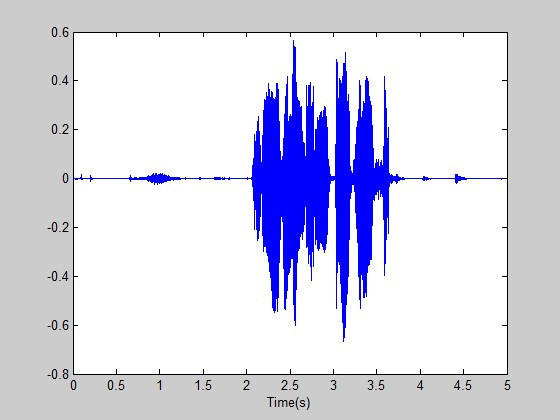图2.1 语音信号时域图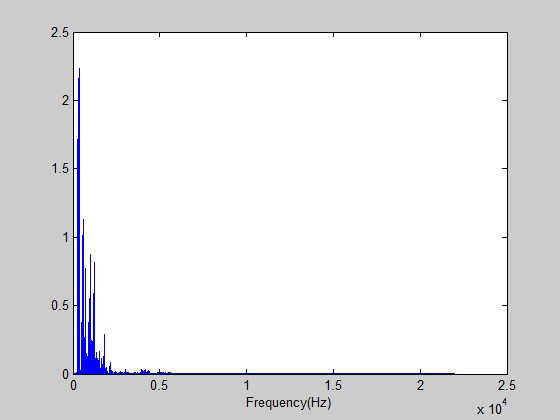图2.2 语音信号频域分析
2.2语音信号的采集
2.2.1语音信号的量化编码采样
在将语音信号进行数字化前，必须先进行防混叠预滤波，预滤波的目的有两个，一是抑制输入信导各领域分量中频率超出/2的所有分量(为采样频率)，以防止混叠干扰；二是抑制50Hz的电源工频干扰。这样，预滤波器必须是一个带通滤波器，设其上、下截止颜率分别是和，则对于绝人多数语音编译码器，=3400Hz、＝60~100Hz、采样率为＝8kHz；而对于语音识别而言，当用于电话用户时，指标与语音编译码器相同。当使用要求较高或很高的场合时＝4500Hz或8000Hz、＝60Hz、＝10kHz或20kHz。
为了将原始模拟语音信号变为数字信号，必须经过采样和量化两个步骤，从而得到时间和幅度上均为离散的数字语音信号。采样也称抽样，是信号在时间上的离散化，即按照一定时间间隔△t在模拟信号x(t)上逐点采取其瞬时值。采样时必须要注意满足奈奎斯特定理，即采样频率必须以高于受测信号的最高频率两倍以上的速度进行取样，才能正确地重建波它是通过采样脉冲和模拟信号相乘来实现的。
在采样的过程中应注意采样间隔的选择和信号混淆：对模拟信号采样首先要确定采样间隔。如何合理选择△t涉及到许多需要考虑的技术因素。一般而言，采样频率越高，采样点数就越密，所得离散信号就越逼近于原信号。但过高的采样频率并不可取，对固定长度（T）的信号，采集到过大的数据量（N=T/△t），给计算机增加不必要的计算工作量和存储空间；若数据量（N）限定，则采样时间过短，会导致一些数据信息被排斥在外。采样频率过低，采样点间隔过远，则离散信号不足以反映原有信号波形特征，无法使信号复原，造成信号混淆。根据采样定理，当采样频率大于信号的两倍带宽时，采样过程不会丢失信息，利用理想滤波器可从采样信号中不失真地重构原始信号波形。量化是对幅值进行离散化，即将振动幅值用二进制量化电平来表示。量化电平按级数变化，实际的振动值是连续的物理量。具体振值用舍入法归到靠近的量化电平上。
语音信号经过预滤波和采样后，由A/D变换器变换为二进制数字码。这种防混叠滤波通常与模数转换器做在一个集成块内，因此目前来说，语音信号的数字化的质量还是有保证的。市面上购买到的普通声卡在这方面做的都很好，语音声波通过话筒输入到声卡后直接获得的是经过防混叠滤波、A/D变换、量化处理的离散的数字信号。
2.2.2利用Windows录音器采集语音信号
在本次设计中，可以利用Windows自带的录音机录制语音文件，图2.3是基于PC机的语音信号采集过程，声卡可以完成语音波形的A/D转换，获得WAV文件，为后续的处理储备原材料。调节录音机保存界面的“更改”选项，可以存储各种格式的WAV文件。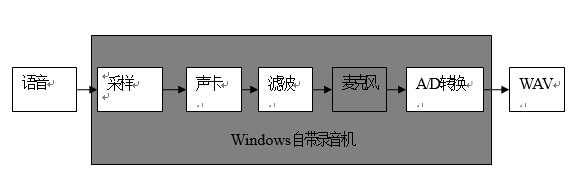图2.3 基于PC机的语音采集过程
第三章 语音信号的分析3.1 语音信号的短时能量分析
一定时宽的语音信号，其能量的大小随时间有明显的变化。清音信号和浊音信号之间的能量差别相当显著。其中清音段（以清音为主要成份的语音段），其能量比浊音段小得多。因此，对语音的短时能量进行分析，可以描述语音的这种特征变化情况。定义短时能量为如式（3-1）所示。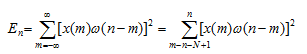（3-1）
其中N为窗长。特殊地，当采用矩形窗时，可简化为如式（3-2）所示。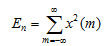（3-2）
也可以从另外一个角度来解释。令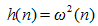（3-3）
则 可表示为如式（3-4）所示。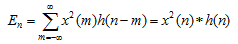（3-4）
可以理解为，首先语音信号各个样点值平方，然后通过一个冲击响应为h(n)的滤波器，输出为由短时能量构成的时间序列。
短时能量的计算直接受冲击响应的选择即窗函数的选择的影响。如果冲击响应的幅度是恒定的，它的序列长度N（即窗长）会很长，将其等效为非常窄的低通滤波器，这时冲击响应对 产生的平滑的作用比较明显，使短时能量基本没有很大的变化，将不能表现出语音的时变的特性。相反，如果冲击响应的序列长度过于小，等效窗就不能提供出够用的平滑，以导致语音的振幅在瞬时的变化的许多细节仍被留了下来，进而不能看出振幅包络变化的规律，一般我们要求窗长是几个基音周期的数量级。
图3.1为采样率8000kHZ，16位，单声道的一个语音信号（单词“earth”）在不同矩形窗长时的短时能量函数，我们会发现：语音信号的幅度变化在被短时能量所反映时，窗长的长短都有影响。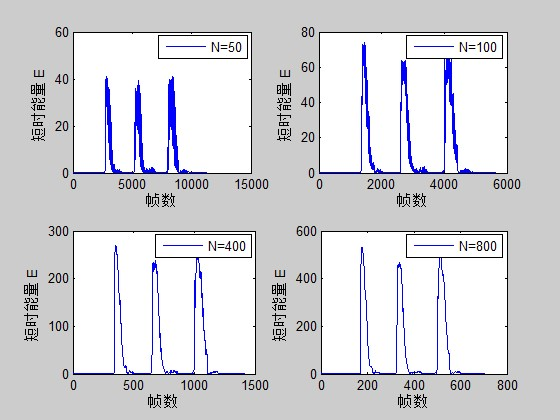图3.1 不同矩形窗长的短时能量函数
我们知道，单词earth前半部分是浊音，后半部分是清音。由以上分析结果可知，浊音部分的能量较之清音部分要大得多，而清音部分的能量相当小，几乎为零。
对语音信号进行短时能量函数运算，可实现以下三点应用：
（1）可用于区分清音段与浊音段。En值大对应于浊音段，En值小对应于清音段。
（2）可用于区分浊音变为清音或清音变为浊音的时间（根据En值的变化趋势）。
（3）对高信噪比的语音信号，也可以用来区分有无语音（语音信号的开始点或终
止点）。无信号（或仅有噪声能量）时，En值很小，有语音信号时，能量显著增大。

3.2短时自相关分析
对于确定性信号序列，自相关函数定义如式（3-5）所示。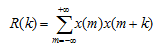（3-5）
对于随机性信号序列或周期性信号序列，自相关函数的定义如式（3-6）所示。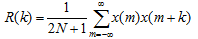（3-6）
自相关函数具有以下几项性质：
(1)若序列是周期性的，假设序列周期为 ，那么其自相关函数也是具有相同周期的周期函数，即
(2)自相关函数是偶函数，即R(k)=R(-k)；
(3)当k=0时，自相关函数有极大值，即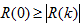；
(4)R(0)为随机性序列的平均功率或确定性信号序列的能量。
自相关函数的上述性质，完全可以适用于语音信号的时域分析中。例如，浊音语音波形序列具有周期性，因此可用自相关函数求出这个周期，即是基音周期。此外，自相关函数也可用在语音信号的线性预测分析中。
短时自相关函数的定义如式（3-7）所示。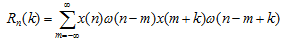（3-7）
令 ，并且 ，可以得到如下式子，如（3-8）所示。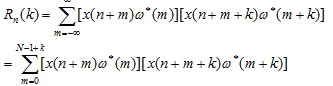（3-8）
如图3.2是在不同的矩形窗窗长条件下单词earth的语音自相关的函数的波形。
对两图分析可得：清音信号的短时自相关函数的波形不具有周期性，也没有明显的峰值，且随着延时k的增大迅速变小，因此其接近于随机噪声；浊音是具有周期性的信号，浊音信号的周期为自相关函数的周期，由此可知，语音信号的性质是浊音还是清音，如果是浊音，还可以得出它的基音周期，它的基音周期可由自相关函数波形中的第一个峰值的位置来估计。所以，自相关函数常用作一下两种作用：
(1) 区分语音信号是清音还是浊音；
(2) 估计浊音语音信号的基音周期。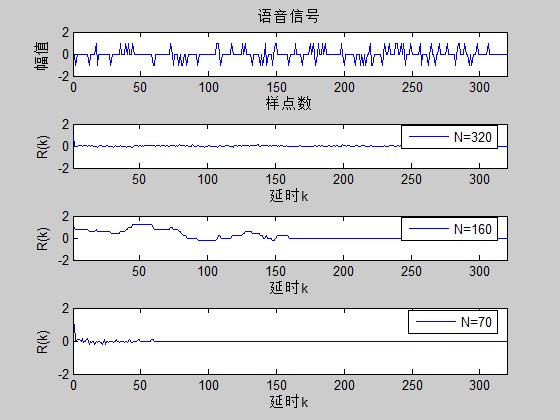图3.2 不同的矩形窗窗长下短时自相关

第四章 语音合成4.1 语音合成技术概述
4.1.1 语音合成技术的意义
语音合成技术涉及声学、语言学、数字信号处理技术、多媒体技术等多个领域, 是当今世界强国竞相研究的热门技术之一。语音合成技术可分为参数合成和波形拼接两种方法。早期的研究主要是采用参数合成方法, 它是计算发音器官的参数, 从而对人的发音进行直接模拟。语音合成已经在许多方面得到了实际应用，方便了人们的生活，创造了很好的经济效益和社会效益，如公共交通中的自动报站、各种场合的自动报警、电话自动查询服务、文本校对中的语音提示等。
本文主要利用载波调制技术进行语音合成。基于载波调制的语音信号合成是以语音信号处理技术、数字信号处理技术为基础，依托于电子计算机、Windows操作系统、MATLAB处理软件等工具将两个信号合成为一个信号。具有较强的实用性、可操作性等特点。
4.1.2 基于载波调制语音合成的基本原理
语音信号合成是一个“分析—存储—合成”的过程。一般是选择合适的基本单元，将基本单元用一定的参数编码方式或波形方式进行存储，形成一个语音库。合成时，根据待合成语音信号，从语音库中取出基本单元进行合成，并将其还原成语音信号。在语音合成中，为了便于存储和后续分析，必须先将语音信号进行预分析、预处理、波形变换等一系列操作。其中，基元是语音合成处理的最小单元，待合成的语音库就是所有语音基元的某中集合。根据基元的选择方式以及其存储形式的不同，可以将合成方法笼统的分为波形合成方法和参数合成方法。
波形合成是一种相对简单的语音合成技术。它把人的发音波形直接存储或者进行进行简单的波形编码后存储，组成一个合成的语音库；合成时，根据待合成的信息，在语音库中取出相应单元的波形数据，拼接或编辑到一起，经过解码还原成语音。该语音合成技术具有一定的局限和不足，但对语音信号具有数据量庞大的特点，这种误差在某种范围内是可以接受的。
基于载波调制的语音信号合成是基于信号的振幅调制原理而实现的。将低频信号加载到高频载波信号的过程，或者说把信息加载到信息载体上以便传输的处理过程，称为调制。所谓“加载”，其实质是使高频载波信号（信息载体）的某个特性参数随信息信号幅值的大小程线性变化。基于载波调制的语音信号合成是以语音一信号作为调制信号，语音二信号为载波信号来进行合成一种以语音二信号声色表述语音一内容的新信号。这种调制方式是用传递的低频信号（如代表语言、音乐、图像的电信号）去控制作为传送载体的高频振荡波（称为载波）的幅度，是已调波的幅度随调制信号的大小线性变化，而保持载波的角频率不变。
4.2 基于载波调制的语音合成基本知识
4.2.1 关键函数希尔伯特变换介绍
本文语音合成的设计思路是用一个语音信号的包络去调制另一个语音信号，实现语音的合成。这就用到了一个关键的函数，希尔伯特变换。在数学与信号处理的领域中，一个实值函数的希尔伯特变换是将信号s(t)与1/(πt)做卷积，以得到s'(t)。因此，希尔伯特变换结果s'(t)可以被解读为输入是s(t)的线性时不变系统的输出，而此系统的脉冲响应为1/(πt)。这是一项有用的数学工具，用在描述一个以实数值载波做调制的信号之复数包络，出现在通讯理论中发挥着重要作用。
希尔伯特变换的频域数学表达式如式（4-1）所示。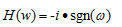（4-1）
其中F是傅里叶变换，i是虚数单位，ω是角频率。
希尔伯特变换等效于 π/2的相移，对正频率产生-π/2的相移，对负频率产生π/2相移，或者说，在时域信号每一频率成分移位1/4波长，因此，希尔伯特变换又称为90度移相器。
MATLAB提供了计算Hilbert变换的函数，其格式为y=Hilbert(x)。但需注意的是，该函数计算出的结果是序列的解析信号，其虚部才是序列的Hilbert变换。
希尔伯特变换在语音信号处理中具有两个性质： 序列x(n)通过Hilbert变换器后，信号频谱的幅度不发生变化，这是因为Hilbert变换器是全通滤波器，引起频谱变化的只是其相位； 序列x(n)与其Hilbert变换是正交的。
4.2.2 信号调制
所谓调制，就是将调制信号加载在三个参数中的某一个参数上，或幅值、或频率、或相位，随调制信号大小成线性或非线性变化的过程。主要有三种基本调制方法，第一种是把调制信号加载在载波信号的幅值上，称为幅度调制 ，简称AM；第二种是把调制信号加载在载波的频率上，称为频率调制，简称FM。 第三种是把调制信号装载在载波的相位上，称为相位调制，简称PM。 本设计采用的是第一种方法，用采集到的语音二信号去对语音一信号进行幅度调制，实现语音合成的目的。
采用调幅调制是因为其以下特点在语音信号处理中得到很好的应用。一是调幅波的振幅（包络）随调制信号变化，而且包络的变化规律与调制信号波形一致，表明调制信号（信息）记载在调幅波的包络中；二是调制系数反应了调幅的强弱程度，一般情况下，调制系数越大调幅度越深。
当调制系数为0时，表示未调幅，即无调幅作用；
当调制系数为1时，此时包络的振幅最小值为0；
当调制系数大于1时，已调波的包络与调制信号不一样，产生严重的包络失真，称为过量调幅。
4.3 语音信号合成过程
4.3.1 语音信号合成流程图
用MATLAB 处理音频信号的基本流程是：先将WAV 格式音频信号经wavread 函数转换MATLAB 列数组变量；再用MATLAB 强大的运算能力进行数据分析和处理，如时域分析、频域分析、数字滤波、信号合成、信号变换、识别和增强等等；处理后的数据如是音频数据，则可用wavwrite函数 转换成WAV 格式文件或用sound、wavplay 等函数直接回放。本设计的语音合成流程图如图4.1所示。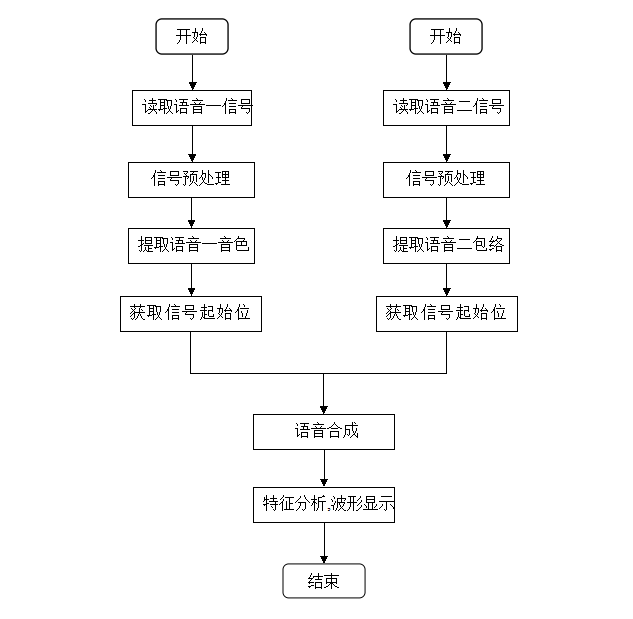图4.1  语音信号合成流程图
4.3.2 语音信号的采集
分析和处理音频信号，首先要对声音信号进行采集，MATLAB 的数据采集工具箱提供了一整套命令和函数，通过调用这些函数和命令，可直接控制声卡进行数据采集。Windows 自带的录音机程序也可驱动声卡来采集语音信号，并能保存为WAV 格式文件，供MATLAB 相关函数直接读取、写入或播放。本文以WAV 格式音频信号作为分析处理的输入数据。
4.3.3 语音信号的合成
声音信号是一种非平稳信号，如果采用简单的时变系统的分析方法，将会产生很大的失真，但是在一小段时间内声音信号完全可以视为平稳信号。因此必须对语音信号做预处理。在本次语音信号合成中采用加窗截断，分帧处理将非平稳信号近似转换为平稳信号。
声音信号特征量提取。声音信号特征量提取包括语音一声音信号声色（频率）的提取和语音二声音信号包络的提取。语音二声音信号包络的提取采用希尔伯特变换实现，得到语音二声音信号的复数包络。
获取语音信号起始位置。在录音过程中控制两段声音从相同的起点开始录取并不是一件容易的事，但是如果不确定语音信号的起始位置直接对语音信号进行合成既存在数据量大又会带来较大的误差。本设计语音合成中拟定连续四个时间点的语音信号强度不为0，则认为语音信号开始，也即找到信号起始位置。
语音信号合成。语音信号合成即是一个调幅载波的过程，是以语音一信号作为载波信号，语音二信号包络作为调幅信号来实现语音合成。实际的载波是一个物理可实现的复杂过程，本语音合成中采用语音一信号点乘包络信号，实际的载波是一个物理可实现的复杂过程，并非简单地乘积运算，然而，得到的合成声音信号效果并不理想，但其波形仍能反应载波过程的实质。
4.4 语音信号合成结果及分析
4.4.1 语音信号预处理结果及分析
该处理过程以语音一信号和语音二声音信号为分析样本。使用Windows系统自带录音器分别录下语音一和语音二，分别命名为one和two,保存为WAV格式。通过MATLAB对所录语音进行采样，采样频率 为16000Hz，获取语音信号并进行加窗。语音一和语音二的时域波形图如图4.2所示，时域图反映出了语音信号的非平稳性。
对采集到的语音信号分别做傅里叶变换进行频谱分析，并显示频谱图，观察各自的幅频谱特性。语音一和语音二的声音信号幅频特性如图4.3所示，语音一和语音二的声音FFT图如图4.2和图4.3。该频谱图横坐标并未进行对应关系处理，但仍不失其频谱特性的本质，由频谱图可清楚地看到样本声音主要以低频为主。人的语音信号频率一般集中在1kHz之前，从声音频谱的包络来看, 根据采样定理，信号宽度近似取为1kHz，重放语音后仍可较清晰的听出原声, 不存在声音混叠现象。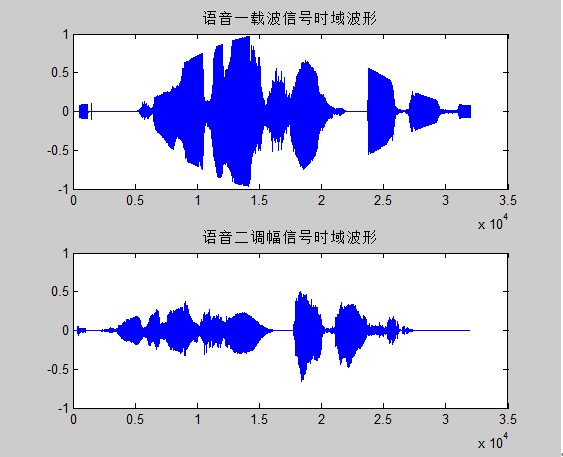图4.2  信号预处理之后时域图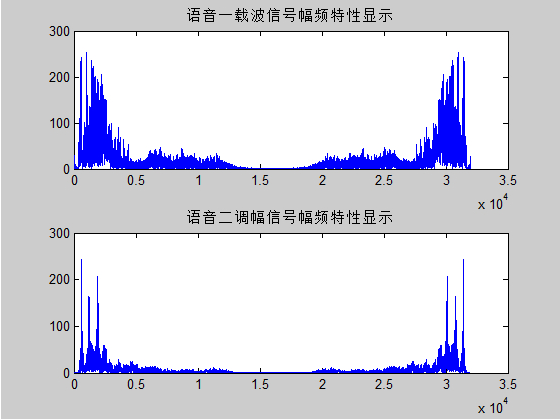图4.3 信号预处理之后频域图
4.4.2 合成语音信号结果及分析
合成语音信号的实质是用语音二信号的包络调制语音一信号振幅的结果。语音二信号包络提取结果如图4.4，该图是语音二信号经希尔伯特—黄变换的虚部显示，因为希尔伯特—黄变换是一个时域信号与1/（πt）的卷积，其结果是载波做调制信号之复数包络，必然蕴含虚部成分，取其虚部的结果必然与时域信号有着直观上的差别，但仍是信号的包络成分。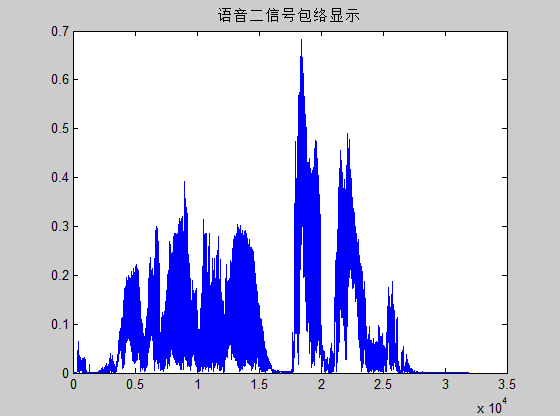图4.4 语音二信号包络图
合成信号的时域显示结果如图4.5所示，该合成信号是以语音一信号的特性和语音二信号的幅度变化的，由其快速傅里叶变换的结果更证实了这一点，其幅频特性与语音一信号的幅频特性更接近。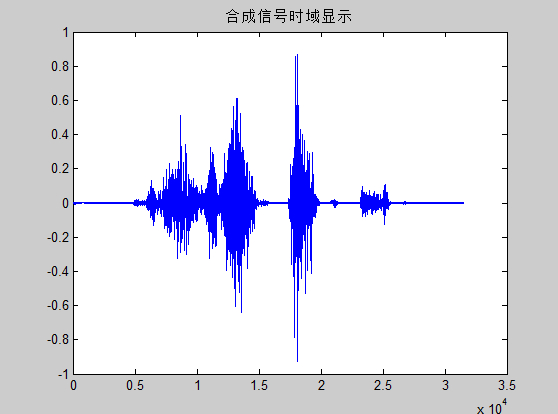图4.5 合成语音信号的时域波形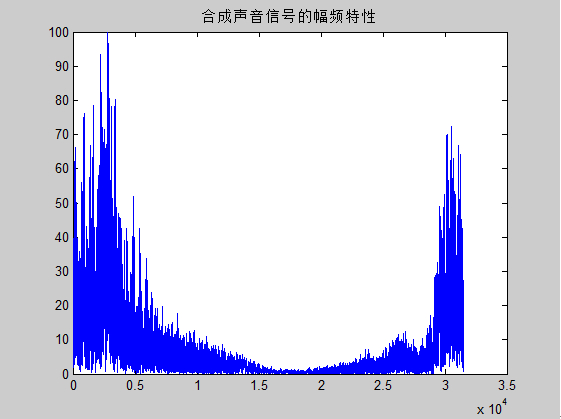图4.6 合成语音信号的幅频特性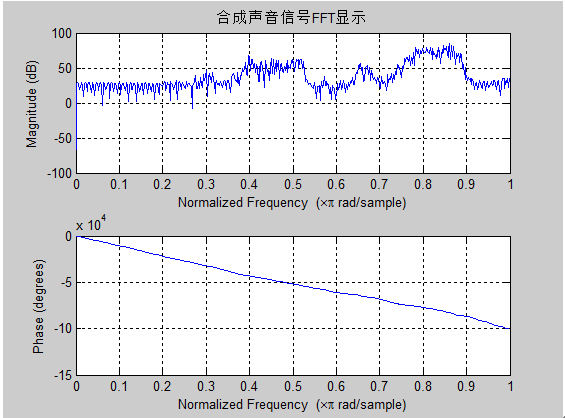图4.7 合成语音信号快速傅里叶变换结果

结  论        随着语音技术的逐渐成熟,语音信号处理技术也在不断发展,不断完善。本文主要研究了通过对语音信号短时能量、短时自相关等特性参数的分析，使我进一步了解了语音信号的特性，明白了只有准确分析并提取出语音信号的特征参数，才能够利用这些参数进行语音编码、语音合成等处理。另外在语音处理方面，我选择了语音合成这一处理方式。基于载波调制的语音处理实现简单，运用广泛，研究这一语音合成方法及特性，对于更加深入地进行各种语音处理有着重要的意义。这次设计我是通过了MATLAB这一平台，MATLAB软件以其强大的运算能力可以很好的完成对语音信号的处理，因此，近一步的加强对MATLAB的研究对我以后的学习会起到很大的帮助。
至此，设计基本符合要求。但是由于个人能力的有限，采集的语音信号清、浊音区分不明显，导致对语音进行短时自相关得出的波形特征不明显。考虑解决方案是通过专业的设备采集语音信号。除此之外，本设计必有其他欠妥之处，请各位老师给予指正！

参考文献 张雪英.数字语音处理[M].北京:电子工业出版社, 2010.
 郑君里,应启绗,杨为理.信号与系统[M].北京:高等教育出版社,2000.
 薛年喜.MATLAB在数字信号处理中的应用[M].北京:清华大学出版社,2003.
 胡航.语音信号处理[M].哈尔滨:哈尔滨工业大学出版社, 2000.
 易克初,田斌,付强.语音信号处理[M].北京:国防工业出版社, 2000.
 万永革.数字信号处理的MATLAB实现[M].北京:科学出版社,2007.
 刘卫国. MATLAB程序设计与应用[M].北京:高等教育出版社,2006.
王嘉梅.基于MATLAB的数字信号处理与时间开发[J].西安:西安电子科技大学出版社,2007:10-14.
 程佩青.数字信号处理教程（第二版）[M].北京:清华大学出版社,2010.
 韩纪庆 张磊 郑铁然.语音信号处理[M].北京:清华大学出版社,2004.
 徐明远,邵玉斌. Matlab仿真在通信与电子工程中的应用[M].西安:西安电子科技大学出版社,2005.
 邓华. Matlab通信仿真及应用实例详解[M].北京:人民邮电出版社,2005.
 张照明,刘政波,刘斌等. 应用Matlab实现信号分析处理[C].北京:科学出版社,2006.
 徐守时. 信号与系统理论方法和应用[M].合肥:中国科学技术大学出版,1999.

 高俊斌. Matlab语言与程序设计[M].武汉:华中理工大学出版社,1998.
附  录
附录A   语音信号特性分析程序
%语音信号时域频域显示%
y=y(:,1);%取单声道
sigLength=length(y);
Y=fft(y,sigLength);
Pyy=Y.* conj(Y) / sigLength;
halflength=floor(sigLength/2);
f=Fs*(0:halflength)/sigLength;
figure;plot(f,Pyy(1:halflength+1));
xlabel('Frequency(Hz)');
t=(0:sigLength-1)/Fs;
figure;
plot(t,y);
xlabel('Time(s)');

%语音信号短时能量%
%x=fscanf(fid,'% f');
%fclose(fid);
s=fra(50,25,x)
s2=s.^2;
energy=sum(s2,2)
subplot(2,2,1)
plot(energy);
xlabel('帧数')
ylabel('短时能量 E')
legend('N=50')
%axis({0,1500,0,10*10^5})
s=fra(100,50,x)
s2=s.^2;
energy=sum(s2,2)
subplot(2,2,2)
plot(energy);
xlabel('帧数')
ylabel('短时能量 E')
legend('N=100')
%axis({0,750,0,2*10^6})
s=fra(400,200,x)
s2=s.^2;
energy=sum(s2,2)
subplot(2,2,3)
plot(energy);
xlabel('帧数')
ylabel('短时能量 E')
legend('N=400')
%axis({0,190,0,7*10^6})
s=fra(800,400,x)
s2=s.^2;
energy=sum(s2,2)
subplot(2,2,4)
plot(energy);
xlabel('帧数')
ylabel('短时能量 E')
legend('N=800')
%axis({0,95,0,14*10^6})

%语音信号短时自相关%
s1=x(1:320);
N=320;   %选择的窗长，加N=320的矩形窗
A=[];
for k=1:320;
sum=0;
for m=1:N-(k-1);
sum=sum+s1(m)*s1(m+k-1);   %计算自相关
end
A(k)=sum;
end
for k=1:320
A1(k)=A(k)/A(1);        %归一化A（k）
end
N=160;                  %选择的窗长，%加N=160的矩形窗
B=[];
for k=1:320;
sum=0;
for m=1:N-(k-1);
sum=sum+s1(m+k-1);   %计算自相关
end
B(k)=sum;
end
for k=1:320
B1(k)=B(k)/B(1);      %归一化B（k）
end
N=70;                 %选择的窗长，加N=70的矩形窗
C=[];
for k=1:320;
sum=0;
for m=1:N-(k-1);
sum=sum+s1(m)*s1(m+k-1);        %计算自相关
end
C(k)=sum;
end
for k=1:320
C1(k)=C(k)/C(1);                %归一化C（k）
end
s2=s1/max(s1)
figure(1)
subplot(4,1,1)
plot(s2)
title('语音信号')
xlabel('样点数')
ylabel('幅值')
axis([0,320,-2,2])
subplot(4,1,2)
plot(A1)
xlabel('延时k')
ylabel('R(k)')
axis([1,320,-2,2]);
legend('N=320')
subplot(4,1,3)
plot(B1);
xlabel('延时k')
ylabel('R(k)')
axis([1,320,-2,2]);
legend('N=160')
subplot(4,1,4)
plot(C1);
xlabel('延时k')
ylabel('R(k)')
axis([0,320,-2,2]);
legend('N=70')
附录B  语音合成主程序
L1=length(y1);                    %测定语音一信号长度
L2=length(y2);                    %测定语音二信号长度
a1=y1.*hamming(L1);               %加窗预处理
a2=y2.*hamming(L2);               %加窗预处理
L1=length(a1);                    %测定语音一信号长度
L2=length(a2);                    %测定语音二信号长度
%采样信号的时域显示
figure(1);
subplot(211);
plot(a1);
title('语音一载波信号时域波形');
subplot(212);
plot(a2);
title('语音二调幅信号时域波形');
%傅里叶频谱绘制
F1=fft(a1,L1);
F2=fft(a2,L2);
AF1=abs(F1);
AF2=abs(F2);
figure(2);
subplot(211);
plot(AF1);
title('语音一载波信号幅频特性显示');
subplot(212);
plot(AF2);
title('语音二调幅信号幅频特性显示');
figure(3);
freqz(F1);
title('语音一载波信号FFT频谱显示');
figure(4);
freqz(F2);
title('语音二载波信号FFT频谱显示');
%获取语音一信号的开始位置
for i=1:L1-4
g(i)=a1(i).*a1(i+1).*a1(i+2).*a1(i+3).*a1(i+4);%认为连续4个幅值不为0的信号即为开始
if g(i)~=0
break;
else i=i+1;
end
end
I=i;

% 获取语音二信号开始位置
for j=1:L2-4
m(j)=a2(j).*a2(j+1).*a2(j+2).*a2(j+3).*a2(j+4);
if m(j)~=0
break;
else j=j+1;
end
end
J=j;
%语音二信号hilbert变换
H=hilbert(a2);
figure(5);
plot(abs(H));
title('语音二信号包络显示');
%信号对齐,语音二包络调制语音一振幅
max1=max(I,J);
for k=1:L1-max1
N(k)=a1(i).*H(j);
i=i+1;
j=j+1;
end
%N=N';
N = N/(max(abs(N)) * 1.05);
wavwrite(N,16000,16,'HC.wav');
figure(6);
plot(imag(N));
title('合成信号时域显示');
pause(1);
sound(10*N,fs);
FN=fft(N);
figure(7);
freqz(FN);
title('合成声音信号FFT显示');
figure(8);
plot(abs(FN));
title('合成声音信号的幅频特性');


展开全文MatLab
• 　我们不讨论如何通过提升仪器精确性和测量方法去减小误差，只讨论数学方案。来看自由式滑雪空中技巧的比赛，对于打分的项目，仅用1个裁判肯定是不靠谱的，再公正的裁判也会不自觉的对自己国家的选手有所偏向，也...二阶近似 泰勒级数 泰勒展开
• 激光干涉仪是精度的线性位移测量仪器，其光波可以直接对米进行定义，可溯源至国家标准。但是我们在使用中往往会出现检测偏离值，偏离我们的预估，以至于在高精度检测时，对设备产生怀疑。今天我们来扒一扒引起激光...
• ## 《单片机原理及应用》复习提纲

万次阅读 多人点赞 2015-12-14 10:42:02
单片机是专门为控制和智能仪器设计的一种集成度很高的微型计算机。 (4) 应用领域 ：   单片机在智能仪表和测量仪器中的应用   单片机在机电/光机电一体化产品中的应用   单片机在计算机...
• 摘 要：介绍了描述传感器静态特性的线性度、重复性、迟滞性和静态误差的定义及计算方法，给出采用计算机加数据采集板卡在Windows系统环境下用VC++编程构成虚拟仪器，实现了对传感器静态特性的自动测试、计算、显示...
• 一般地，仪器仪表主要由传感器部分、测量部分及输出部分组成。 　（1）传感器部分 其作用是将某些物理量，通过传感器转换成相应的电信号，并送入测量电路中。例如：测温仪表的传感器部分是利用传感元件（热电偶、...
• 介绍了采用三次样条曲线插值方法对称重仪表的非线性误差进行修正的方法，该修正方法对于线性误差较大的传感器有很好的补偿效果，在某些测量精度较高的场合采用三次样条曲线插值可以获得比流行的多段折线线性补偿方法...
•   这篇文章详细推导了一元线性回归方程的参数解，供新手朋友参考。   假定一元线性回归方程的具体形式为 y=a+bx(1) y=a+bx \tag{1} y=a+bx(1) 现在，为确定参数a,ba,ba,b进行了nnn次观测，观测结果为： i123⋯...参数估计 最小二乘法
• 摘要介绍TI公司的TLC2543的特性，与51系列单片机的接口以及在仪器仪表中的应用。 　关键词　串行A/D应用 　1引言 　TLC2543是TI公司的12位串行模数转换器，使用开关电容逐次逼近技术...　（6）线性误差±1LSBmax；
• 文章目录 1、锁相环是啥呢？ Q1：啥是鉴相器？ 2、分频器 3、74LS161分配器 4、74LS00...它在大于40dB的增益控制范围内，工作频率为200MHz时，可提供优于±0．5dB的线性误差，而在400MHz时可提供优于±1dB的线性误差
• [《Interpretable Machine Learning》 Christoph Molnar](https://christophm.github.io/interpretable-ml-book/index.html) 内容围绕：对于一个训练好的线性...上图为一个线性回归的单个实例，最后一项为误差。 ...机器学习 可视化 数据可视化 统计模型
• 一般地，仪器仪表主要由传感器部分、测量部分及输出部分组成。 　（1）传感器部分 其作用是将某些物理量，通过传感器转换成相应的电信号，并送入测量电路中。例如：测温仪表的传感器部分是利用传感元件（热电偶、...
• 放大器各级以及放大器输出，与第三级间均存在深度的负反馈，这样不但得到了在小信号时检波二极管的非线性特性，使指示具有良好的线性。 　放大后的信号是交流电，因电极极片间及电极引线，均出现了不可忽视的分布...
• 卡尔曼滤波就是线性最小方差估计 你根据线性最小方差估计就可以推导出卡尔曼滤波那些东西。 下面是截图自我的notability的笔记 下面摘自：https://blog.csdn.net/victor_zy/article/details/82862904 ...
• 放大器各级以及放大器输出，与第三级间均存在深度的负反馈，这样不但得到了在小信号时检波二极管的非线性特性，使指示具有良好的线性。 　放大后的信号是交流电，因电极极片间及电极引线，均出现了不可忽视的分布...
• 经典线性回归方法假设变量 X 和 Y 是正态分布的，并且在浓度范围内具有测量误差。 然而，在方法比较研究中，我们通常发现测量值的分布不正常，误差的方差不是一致的。 Passing & Bablok 回归不需要对预期值或误差项...matlab
• ## 误差与残差

千次阅读 2018-09-14 09:33:00
这种真实值（true value）往往是不可观测的，比如用仪器去测量一个物体的长度，无论是采用简单的直尺，还是采用高精度的游标卡尺，亦或是螺旋测微器，都无法观测到直尺的真实长度。观测值只能无限靠近真实值，却......